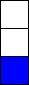You may also likeCubes

How many faces can you see when you arrange these three cubes in different ways?Pebbles

Place four pebbles on the sand in the form of a square. Keep adding as few pebbles as necessary to double the area. How many extra pebbles are added each time?Bracelets

Investigate the different shaped bracelets you could make from 18 different spherical beads. How do they compare if you use 24 beads?

Matching Fractions, Decimals and Percentages

Age 7 to 14 Challenge Level:

One third is the number you get when you divide one by three.
One third can be represented in all the following different ways:$$\frac{1}{3} \mbox{ or as an equivalent fraction e.g. } \frac{3}{9} \mbox{ or } \frac{5}{15}$$

$$0.3333... = 0.3 \mbox{ recurring } = 0.\dot{3}$$

$$33 \frac{1}{3}\text{ out of }100 = 33 \frac{1}{3} \%$$